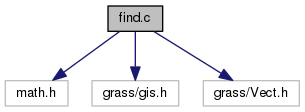GRASS Programmer's Manual  6.5.svn(2014)-r66266
vector/Vlib/find.c File Reference
`#include <math.h>`
`#include <grass/gis.h>`
`#include <grass/Vect.h>`
Include dependency graph for vector/Vlib/find.c:Go to the source code of this file.

## Macros

#define HUGE_VAL   9999999999999.0

## Functions

int Vect_find_node (struct Map_info *Map, double ux, double uy, double uz, double maxdist, int with_z)
Find the nearest node. More...

int Vect_find_line (struct Map_info *map, double ux, double uy, double uz, int type, double maxdist, int with_z, int exclude)
Find the nearest line. More...

int Vect_find_line_list (struct Map_info *map, double ux, double uy, double uz, int type, double maxdist, int with_z, struct ilist *exclude, struct ilist *found)
Find the nearest line(s). More...

int Vect_find_area (struct Map_info *Map, double x, double y)
Find the nearest area. More...

int Vect_find_island (struct Map_info *Map, double x, double y)
Find the nearest island. More...

## Macro Definition Documentation

 #define HUGE_VAL   9999999999999.0

Definition at line 23 of file vector/Vlib/find.c.

Referenced by Vect_find_line_list(), and Vect_find_node().

## Function Documentation

 int Vect_find_area ( struct Map_info * Map, double x, double y )

Find the nearest area.

Parameters
 [in] Map vector map [in] x,y point coordinates
Returns
area number
0 if not found

Definition at line 248 of file vector/Vlib/find.c.

 int Vect_find_island ( struct Map_info * Map, double x, double y )

Find the nearest island.

Parameters
 [in] Map vector map [in] x,y points coordinates
Returns
island number,
0 if not found

Definition at line 295 of file vector/Vlib/find.c.

 int Vect_find_line ( struct Map_info * map, double ux, double uy, double uz, int type, double maxdist, int with_z, int exclude )

Find the nearest line.

Parameters
 [in] map vector map [in] ux,uy,uz points coordinates [in] type feature type (GV_LINE, GV_POINT, GV_BOUNDARY or GV_CENTROID) if only want to search certain types of lines or -1 if search all lines [in] maxdist max distance from the line [in] with_z 3D (WITH_Z, WITHOUT_Z) [in] exclude if > 0 number of line which should be excluded from selection. May be useful if we need line nearest to other one.
Returns
number of nearest line
0 if not found

Definition at line 110 of file vector/Vlib/find.c.

Referenced by Vect_net_nearest_nodes(), and Vedit_snap_point().

 int Vect_find_line_list ( struct Map_info * map, double ux, double uy, double uz, int type, double maxdist, int with_z, struct ilist * exclude, struct ilist * found )

Find the nearest line(s).

Parameters
 [in] map vector map [in] ux,uy,uz points coordinates [in] type feature type (GV_LINE, GV_POINT, GV_BOUNDARY or GV_CENTROID) if only want to search certain types of lines or -1 if search all lines [in] maxdist max distance from the line [in] with_z 3D (WITH_Z, WITHOUT_Z) [in] exclude list of lines which should be excluded from selection [in] found list of found lines (or NULL)
Returns
number of nearest line
0 if not found

Definition at line 145 of file vector/Vlib/find.c.

 int Vect_find_node ( struct Map_info * Map, double ux, double uy, double uz, double maxdist, int with_z )

Find the nearest node.

Parameters
 [in] Map vector map [in] ux,uy,uz point coordinates [in] maxdist max distance from the line [in] with_z 3D (WITH_Z, WITHOUT_Z)
Returns
number of nearest node
0 if not found

Definition at line 38 of file vector/Vlib/find.c.

Referenced by Vect_overlay_and().